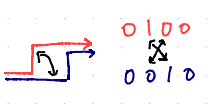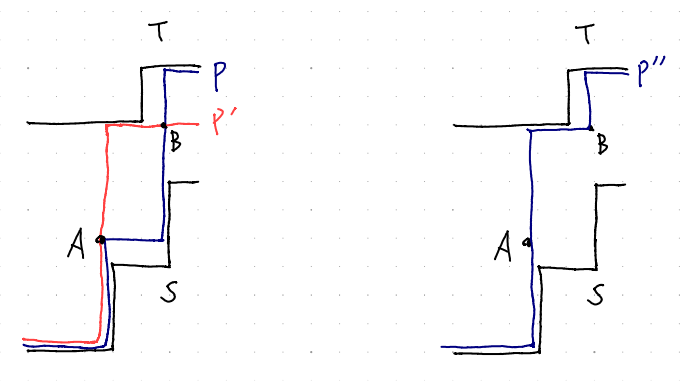Contest Duration: - (local time) (120 minutes) Back to Home

## Solution

When the first character is fixed, the optimal string $$x$$ can be constructed greedily, using the same character as the previous as long as the following condition is satisfied.

• The number of 0s among the first $$i$$ characters of $$x$$ is between that of $$s$$ and that of $$t$$.

Therefore, for each of the two cases where the first character is 0 and where it is 1, we can greedily construct the string and find its beauty, and then we print the greater.

Sample Implementation in C++

## Proof

Below we prove the validity of the greedy strategy.

### Associate a string with a path in a two-dimensional grid

Let us associate a string $$s\in S_{n,m}$$ with a path from the coordinates $$(0, 0)$$ to $$(n, m)$$ in $$n+m$$ steps as follows.

• When we are at $$(x, y)$$ before the $$i$$-th step, go to $$(x+1, y)$$ if the $$i$$-th character of $$s$$ is 0, and go to $$(x, y+1)$$ if that character is 1.

In this path, swapping two adjacent characters in the string corresponds to “folding back” a corner in the path.Thus, if $$S$$ and $$T$$ are the path corresponding to $$s$$ and $$t$$, the path corresponding to a string between $$s$$ and $$t$$ lies between $$S$$ and $$T$$ (in the region surrounded by $$S$$ and $$T$$). Additionally, the beauty is maximized by minimizing the number of turns.

If going straight would take us out of the region between $$S$$ and $$T$$, we need to make a turn. Let us call turns made in other cases bad. It is sufficient to show that we do not need to consider making bad turns when minimizing the number of turns.

We can show this as follows. Let $$P$$ be an arbitrary path from $$(0,0)$$ to $$(n,m)$$ that lies between $$S$$ and $$T$$, and assume that $$P$$ makes a bad turn. By fixing $$P$$ as described below, we can construct a path $$Q$$ that satisfies the following conditions.

• The number of turns is not more than that in $$P$$.
• The first bad turn is made later than in $$P$$ (that is, there are more steps before the first bad turn), or there is no bad turn.

Let $$A$$ be the point where the first bad turn among the $$n+1$$ steps is made. Let $$P'$$ be the path where we do not make a turn at $$A$$ and instead make a turn when hitting $$S$$ or $$T$$. Let $$B$$ be the first point after $$A$$ where $$P$$ and $$P'$$ cross (share the same point). From $$A$$ to $$B$$ (including both ends), $$P'$$ always makes exactly one turn, while $$P$$ makes two or more turns. If $$B=(n,m)$$, $$P'$$ makes no bad turns, so we can let this $$P'$$ be $$Q$$. Otherwise, consider a new path $$P''$$ that traces $$P'$$ until $$B$$ and traces $$P$$ after that. From $$A$$ to $$B$$ (including both ends), $$P''$$ always makes exactly two turns. Additionally, the first bad turn of $$P''$$ is at $$B$$. Thus, we can let this $$P''$$ be $$Q$$.By repeatedly constructing $$Q$$ from $$P$$, we can eventually have a path without bad turns (because as long as the path has a bad turn, it makes the first bad turn later and later, but the path from $$(0, 0)$$ to $$(n, m)$$ has a finite number of steps which is $$n+m$$). This completes the proof.

posted:
last update: